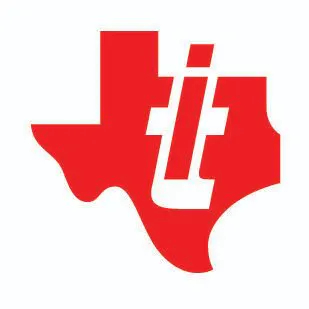Published

# Wood Gas Generator (Energy From Waste)

Generation of wood gas (fuel) using waste around us.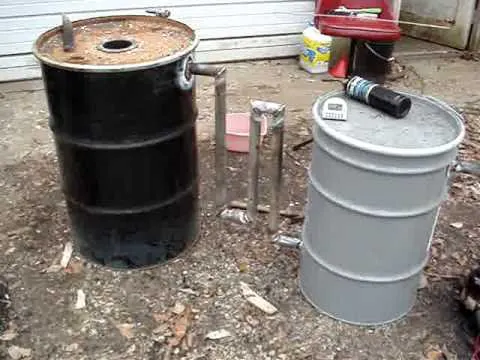## Things used in this project

### Hardware components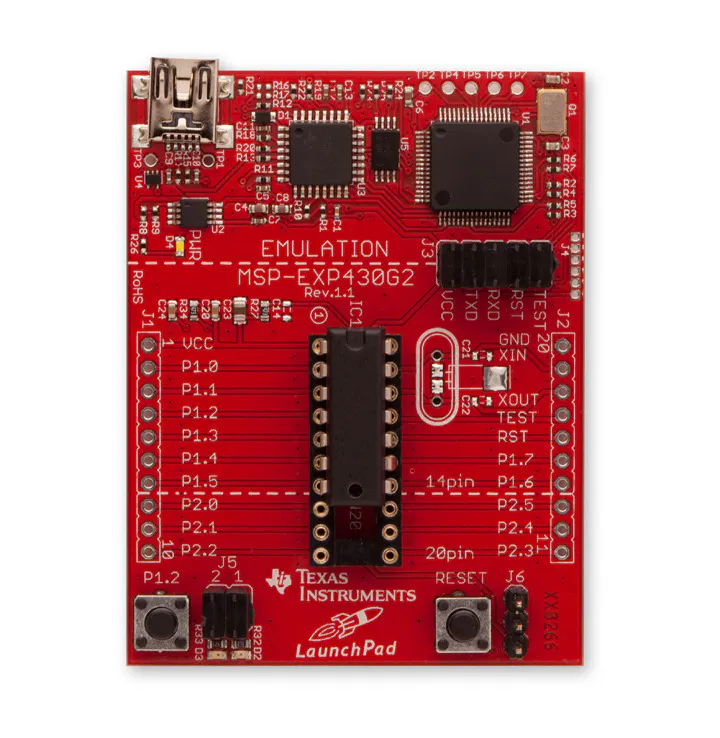×1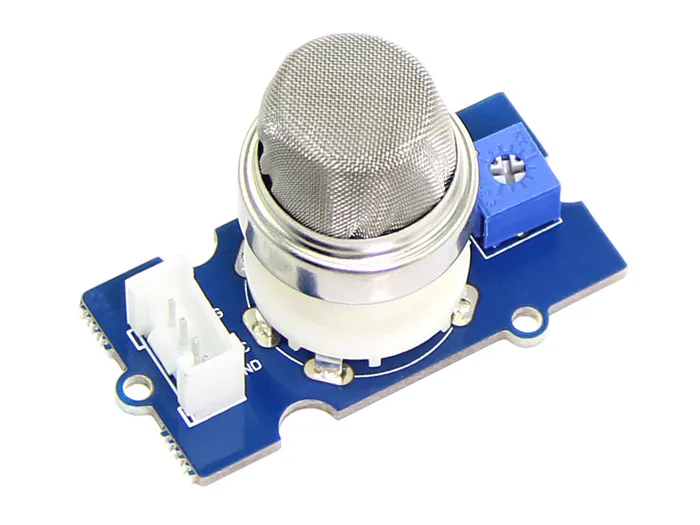Seeed Grove - Gas Sensor(MQ2)
×1

### Software apps and online services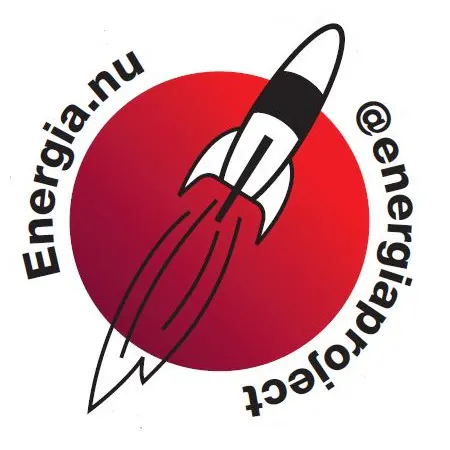Texas Instruments Energia

## Code

### Hydrogen Concentration

C/C++
```const int AOUTpin=0;//the AOUT pin of the hydrogen sensor goes into analog pin A0 of the arduino

int limit;
int value;

void setup() {
Serial.begin(9600);//sets the baud rate
}

void loop()
{
Serial.print("Hydrogen value: ");
Serial.println(value);//prints the hydrogen value
delay(100);
}
```

### Smoke Concentration in PPM

C/C++
```#define         MQ_PIN                       (2)
#define         RL_VALUE                     (5)
#define         RO_CLEAN_AIR_FACTOR          (9.83)
#define         CALIBARAION_SAMPLE_TIMES     (50)
#define         CALIBRATION_SAMPLE_INTERVAL  (500)

#define         GAS_LPG                      (0)
#define         GAS_CO                       (1)
#define         GAS_SMOKE                    (2)

float           LPGCurve  =  {2.3,0.21,-0.47};
float           COCurve  =  {2.3,0.72,-0.34};
float           SmokeCurve = {2.3,0.53,-0.44};
float           Ro =  10;

void setup()
{
Serial.begin(9600);
Serial.print("Calibrating...\n");
Ro = MQCalibration(MQ_PIN);
Serial.print("Calibration is done...\n");
Serial.print("Ro=");
Serial.print(Ro);
Serial.print("kohm");
Serial.print("\n");
}

void loop()
{
Serial.print("LPG:");
Serial.print( "ppm" );
Serial.print("    ");
Serial.print("CO:");
Serial.print( "ppm" );
Serial.print("    ");
Serial.print("SMOKE:");
Serial.print( "ppm" );
Serial.print("\n");
delay(500);
}

{
}

float MQCalibration(int mq_pin)
{
int i;
float val=0;
for (i=0;i<CALIBARAION_SAMPLE_TIMES;i++) {
delay(CALIBRATION_SAMPLE_INTERVAL);
}
val = val/CALIBARAION_SAMPLE_TIMES;
val = val/RO_CLEAN_AIR_FACTOR;
return val;
}

{
int i;
float rs=0;
}
return rs;
}

int MQGetGasPercentage(float rs_ro_ratio, int gas_id)
{
if ( gas_id == GAS_LPG ) {
return MQGetPercentage(rs_ro_ratio,LPGCurve);
} else if ( gas_id == GAS_CO ) {
return MQGetPercentage(rs_ro_ratio,COCurve);
} else if ( gas_id == GAS_SMOKE ) {
return MQGetPercentage(rs_ro_ratio,SmokeCurve);
}
return 0;
}

int  MQGetPercentage(float rs_ro_ratio, float *pcurve)
{
return (powf(10,( ((logf(rs_ro_ratio)-pcurve)/pcurve) + pcurve)));
}
```

## Credits

### Nikhil Nair

1 project • 1 follower